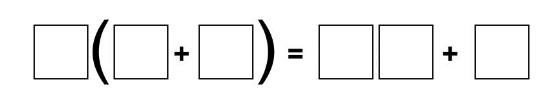Home > Grade 6 > Expressions & Equations > Distributive Property

# Distributive Property

Directions: Using the digits 1 to 9 at most one time each, fill in the boxes to make a true equation.### Hint

What numbers would you need in the parentheses to give you a two digit number and a one digit number when you distribute?

There are many possible answers. One possible answer: 2(9 + 3) = 18 + 6

## Largest Possible GCF #2

Directions: Using the digits 0-9 at most once, fill in the boxes to make the …

1.2(5+4) = 10 + 8

Going to try this with my students after having studied and reviewed the distributive property with algebra tiles. I’ll let you know how it goes!

2.I’m not so sure there are all that many possible answers. I convinced myself (though I’m not 100% sure yet) that there are only 4: the two already listed, and also 2(7+3) = 14 + 6 and 2(5+3)=10 + 6.

•In addition to the 4 that are now listed I also have 4(9+2) = 36 +8 as a solution.
These are the answers I come up with if I want both sides of the equation to simply show distributing and factoring of numbers.
There are, however, more possible answers if we open up the problem to any equivalent statements.
For example: 5(3+4) = 28 + 7
Both sides of the equation equal 35 (if I did my math correctly). I haven’t made a list of all of those possibilities but I imagine there are quite a few more. I have found that it depends on how I frame the problem the types of answers I get. If students know it is to work on distributive property the solutions they come up with are the 5 we have listed. If I don’t mention the distributive property then I get both types of solutions.

•This is such a great observation. As a math educator and designer of professional learning, I personally only “played” with solutions that model distribution/factoring. But during a training today, many teachers generated true equations without representing distribution/factoring. So, I agree – it totally depends on your learning goals for your audience.

However, we thought it would be cool to have students attempt to model all solutions with linking cubes or square tiles to see the difference between these two types of equations. Very neat to think about…

3.Since you can use 0 as a digit, that opens up two more possible answers:
2(4+3) = 08 + 6, and 2(3+4) = 06 + 8

•4.